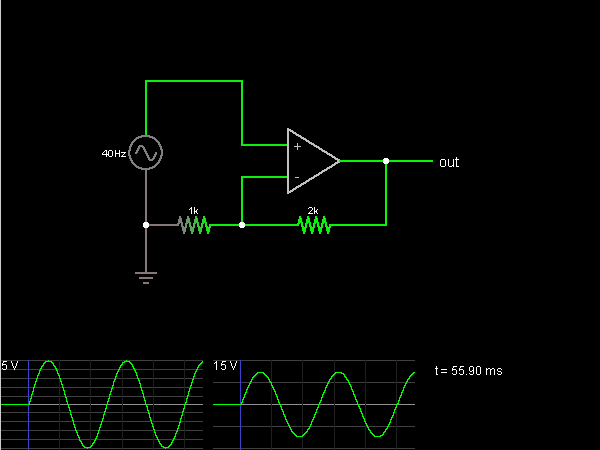# Circuit Simulator - Noninverting Amplifier

## Where can I get Noninverting Amplifier Circuit Diagram with Explanation?

IndiaBIX provides you lots of fully solved Noninverting Amplifier circuit diagram with detailed explanation and working principles.

## How to design a Noninverting Amplifier (electronic circuit)?

You can easily design the Noninverting Amplifier circuit by practicing the exercises given below. Here you can design and simulate your own electronic circuits with this Online Circuit Designer and Simulator.### Circuit Description:

This circuit amplifies the input without inverting it, multiplying the voltage by 3, using an op-amp. The op-amp attempts to keep its two inputs at the same voltage. One is at the input voltage, so for the other one to be the same, there must be a voltage drop across the 1k ohm resistor equal to the input voltage. The 2k ohm resistor has the same current across it so the voltage drop must be twice as large, by Ohm's Law. So the output voltage is equal to the input plus 2x the input, or a total of 3x the input. -- Credits: Mr. Paul Falstad.